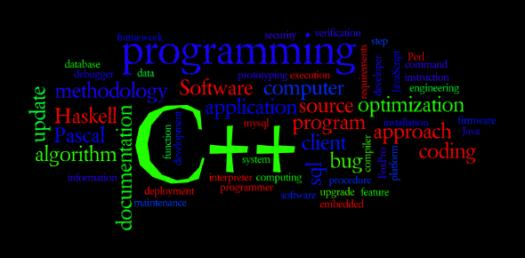# C++ Programming Trivia Questions Test: Can You Pass This Quiz?

20 Questions | Total Attempts: 65SettingsAre you looking for a C++ Programming Trivia Questions Test? The quiz below is perfect for seeing just how much you know about this language and if it is enough to earn you a good score in the certification exam. Can You Pass This Quiz? How about you give it a shot and see how well you will do!

• 1.
Can we have overloaded virtual functions?
• A.

True

• B.

False

• 2.
Is “this” is a reference variable?
• A.

True

• B.

False

• 3.
Which is the highest precedence operator?
• 4.
Oracle engine automatically locks table data while executing SQL statements
• A.

True

• B.

False

• 5.
Is the below syntax is correct?      Exception           when OTHERS THEN              DBMS_OUTPUT.PUT_LINE("ERROR");            WHEN TOO-MANY-ROWS-FOUND THEN              DBMS_OUTPUT.PUT_LINE("MORE ROWS FOUND");
• A.

True

• B.

False

• 6.
Can we define a class inside another class?
• A.

True

• B.

False

• 7.
Static member variables of a class can be initialized by using Constructor?
• A.

True

• B.

False

• 8.
Which is the lowest precedence operator?
• 9.
There is a limit to the number of cursors that can be opened in a session.
• A.

True

• B.

False

• 10.
Can we use a commit statement within a database trigger?
• A.

True

• B.

False

• 11.
Can constructor throw an exception?
• A.

True

• B.

False

• 12.
Can we have duplicate cases in the switch?
• A.

True

• B.

False

• 13.
Can we have virtual constructors?
• A.

True

• B.

False

• 14.
Can we write trigger on views?
• A.

True

• B.

False

• 15.
IN is faster than EXISTS.
• A.

True

• B.

False

• 16.
42 is the ASCII value of '\0'
• A.

True

• B.

False

• 17.
Can we call the destructor explicitly?
• A.

True

• B.

False

• 18.
• A.

True

• B.

False

• 19.
The name of the table can be changed using the ALTER TABLE clause.
• A.

True

• B.

False

• 20.
Check constraint consists of subqueries and sequences.
• A.

True

• B.

False

Related TopicsBack to top